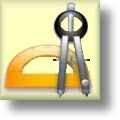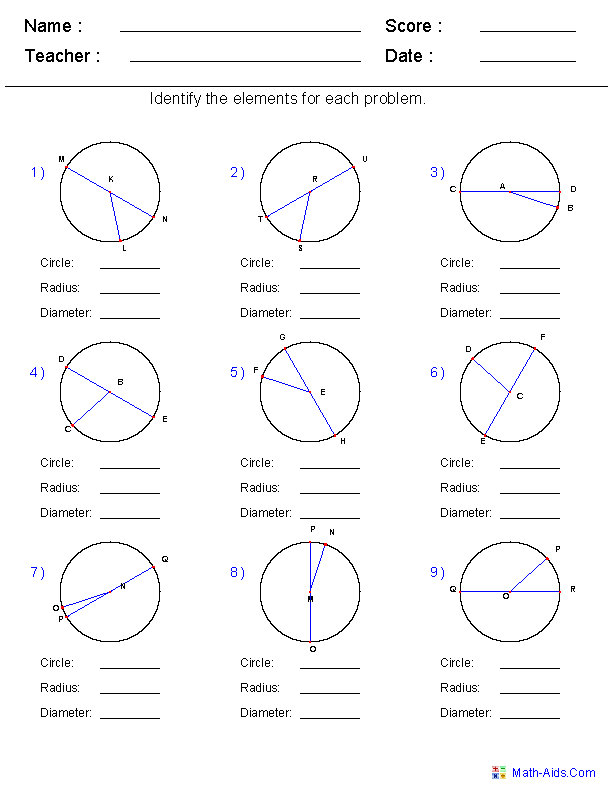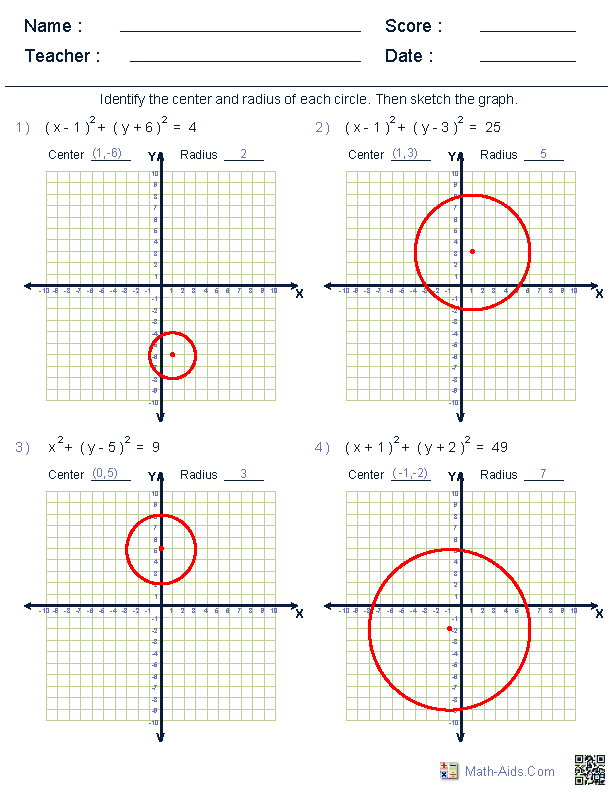#Geometry Worksheets

## Circle Worksheets

Here is a graphic preview for all of the Circle Worksheets. You can select different variables to customize these Circle Worksheets for your needs. The Circle Worksheets are randomly created and will never repeat so you have an endless supply of quality Circle Worksheets to use in the classroom or at home. We have identifying radius and diameter for circles worksheets, calculating circumference, area, radius, and diameters worksheets, arcs and central angles for circles worksheets, arcs and chords worksheets, inscribed angles worksheets, graphing of circles worksheets and much more circle worksheets for your use. Our Circle Worksheets are free to download, easy to use, and very flexible.

## Quick Link for All Circle Worksheets

Click the image to be taken to that Circle Worksheet.

##### Identify Radius & DiameterCircle Worksheets##### Circumference, Area, Radius,& Diameter Worksheets##### Graphing Equation ofCircle WorksheetsRecommended Videos

## Detailed Description for All Circle Worksheets

These Circle Worksheet are great for practicing identifying Circle, Radius, and Diameter from graphs. The worksheet will produce 9 problems per page.

Circumference, Area, Radius, & Diameter Worksheets
These Circle Worksheets are great for practicing solving for the circumference, area, radius and diameter of a circle. The worksheet will produce 9 problems per page.

Graphing Equation of Circle Worksheets
These Circle Worksheets are great for practicing graphing circles from their equations.
The worksheet will produce 9 problems per page.